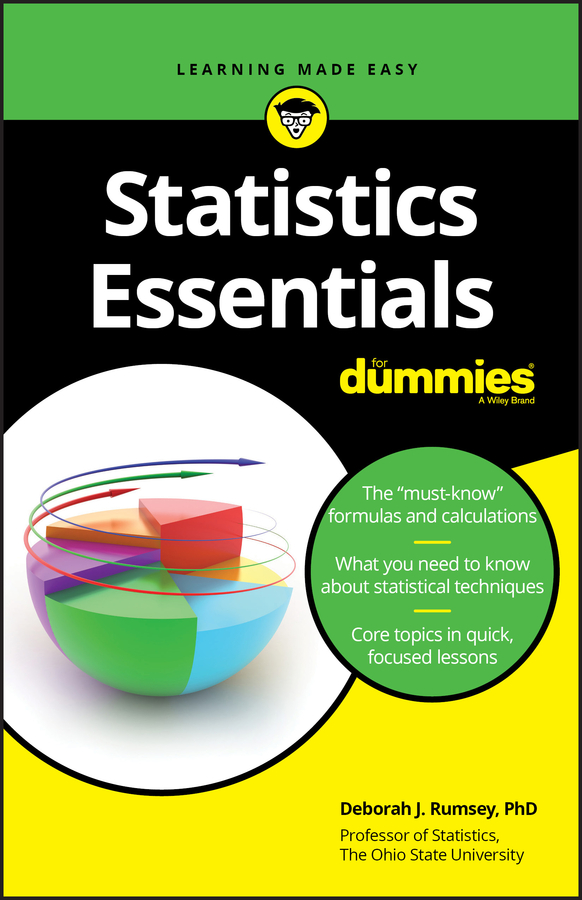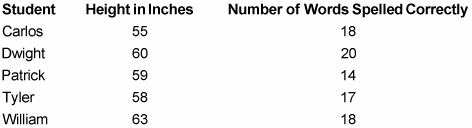##### Statistics Essentials For DummiesQuantitative data assigns a numerical value to each member of a statistical sample. You can use this information to find the mean and median values.

The following sample — which is purely fictional — uses five members of Sister Elena’s basketball team. Suppose that the information in the following list has been gathered about each team member’s height and most recent spelling test.You can use this information to find the mean and median for both sets of data. Both terms refer to ways to calculate the average value in a quantitative data set. An average gives you a general idea of where most individuals in a data set fall so you know what kinds of results are standard. For example, the average height of Sister Elena’s fifth grade class is probably less than the average height of the Los Angeles Lakers. As you will learn here, an average can be misleading in some cases, so knowing when to use the mean versus the median is important.

## How to find the mean

The mean is the most commonly used average. In fact, when most people use the word average, they’re referring to the mean. Here’s how you find the mean of a set of data:
1. Add up all the numbers in that set.

For example, to find the average height of the five team members, first add up all their heights:

55 + 60 + 59 + 58 + 63 = 295

2. Divide this result by the total number of members in that set.

Divide 295 by 5 (that is, by the total number of boys on the team):

295 ÷ 5 = 59

So the mean height of the boys on Sister Elena’s team is 59 inches.

Similarly, to find the mean number of words that the boys spelled correctly, first add up the number of words they spelled correctly:

18 + 20 + 14 + 17 + 18 = 87

Now divide this result by 5:

87 ÷ 5 = 17.4

As you can see, when you divide, you end up with a decimal in your answer. If you round to the nearest whole word, the mean number of words that the five boys spelled correctly is about 17 words.

The mean can be misleading when you have a strong skew in data — that is, when the data has many low values and a few very high ones, or vice versa.

For example, suppose that the president of a company tells you, “The average salary in my company is \$200,000 a year!” But on your first day at work, you find out that the president’s salary is \$19,010,000 and each of his 99 employees earns \$10,000. To find the mean, add up the total salaries:

\$19,010,000 + (99 \$10,000) = \$20,000,000

Now divide this number by the total number of people who work there:

\$20,000,000 ÷ 100 = \$200,000

So the president didn’t lie. However, the skew in salaries resulted in a misleading mean.

## How to find the median

When data values are skewed (when a few very high or very low numbers differ significantly from the rest of the data), the median can give you a more accurate picture of what’s standard. Here’s how to find the median of a set of data:
1. Arrange the set from lowest to highest.

To find the median height of the boys in the above table, arrange their five heights in order from lowest to highest.

55 58 59 60 63

2. Choose the middle number.

The middle value, 59 inches, is the median average height.

To find the median number of words that the boys spelled correctly (see the above table), arrange their scores in order from lowest to highest:

14 17 18 18 20

This time, the middle value is 18, so 18 is the median score.

If you have an even number of values in the data set, put the numbers in order and find the mean of the two middle numbers in the list. For instance, consider the following:

2 3 5 7 9 11

The two center numbers are 5 and 7. Add them together to get 12 and then divide by 2 to get their mean. The median in this list is 6.

Now recall the company president who makes \$19,010,000 a year and his 99 employees who each earn \$10,000. Here’s how this data looks:

10,000 10,000 10,000 ... 10,000 19,010,000

As you can see, if you were to write out all 100 salaries, the center numbers would obviously both be 10,000. The median salary is \$10,000, and this result is much more reflective of what you’d probably earn if you were to work at this company.